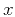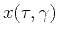Huygens wavefront tracing: A robust alternative to conventional ray tracingNext: A discretization scheme and Up: Sava & Fomel: Huygens Previous: Introduction

# Continuous theory

The eikonal equation, governing the traveltimes from a fixed source in an isotropic heterogeneous medium, has the form(1)

Here,, andare spatial coordinates,is the traveltime (eikonal), andstands for the velocity field. Constant-traveltime contours in the traveltime field, constrained by equation (1) and appropriate boundary conditions, correspond to wavefronts of the propagating wave. Additionally, each point on a wavefront can be parameterized by an arbitrarily chosen ray parameter. In three dimensions,includes a pair of independent parameters. For brevity, from now on we will restrict the analysis to two dimensions. One can easily generalize it to the 3-D case by consideringandas vector quantities. Thus, we will refer to the following two-dimensional form of equation (1):(2)

For a point source,can be chosen as the initial ray angle at the source. Zhang (1993) shows thatas a function of spatial coordinates satisfies the simple partial differential equation(3)

Equation (3) merely expresses the fact that in an isotropic medium, rays are locally orthogonal to wavefronts. The fieldhas not only theoretical interest as it provides one of the possible ways for evaluating propagation amplitudes. In particular, the geometrical spreading factoris connected toby the simple relationship (Zhang, 1993)(4)

It is important to note that for complex velocity fields, bothandas functions ofandbecome multi-valued. In this case, the multi-valued character of the ray parametercorresponds to the situation, where more than one ray from the source passes through a particular pointin the subsurface. This situation presents a very difficult problem when equations (2) and (3) are solved numerically. Typically, only the first-arrival branch of the traveltime is picked in the numerical calculation. The ray tracing method is free from that limitation because it operates in the ray coordinate system. Ray tracing computes the traveltimeand the corresponding ray positionsandfor a fixed ray parameter.

Sinceandare uniquely defined for arbitrarily complex velocity fields, we can now make an important mathematical transformation. Considering equations (2) and (3) as a system and applying the general rules of calculus, we can transform this system by substituting the inverse functionsandfor the original fieldsand. The resultant expressions take the form(5)

and(6)

Comparing equations (5) and (6) with the original system (2-3) shows that equations (5) and (6) again represent the dependence of ray coordinates and Cartesian coordinates in the form of partial differential equations. However, the solutions of system (5-6) are better behaved and have a unique value for everyand. These values can be computed with the conventional ray tracing. However, the ray-tracing approach is based on a system of ordinary differential equations, which represents a different mathematical model.

We use equations (5) and (6) as the basis of our wavefront tracing algorithm. The next section discusses the discretization of the differential equations and the physical interpretation we have given to the scheme.Huygens wavefront tracing: A robust alternative to conventional ray tracingNext: A discretization scheme and Up: Sava & Fomel: Huygens Previous: Introduction

2014-03-11